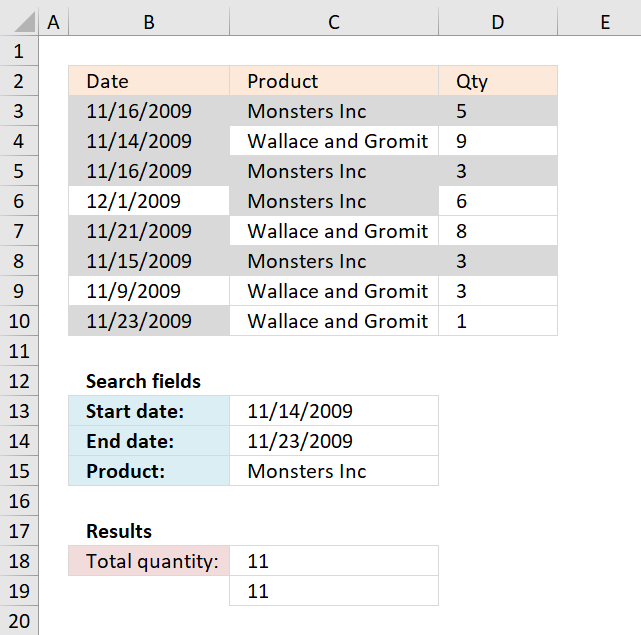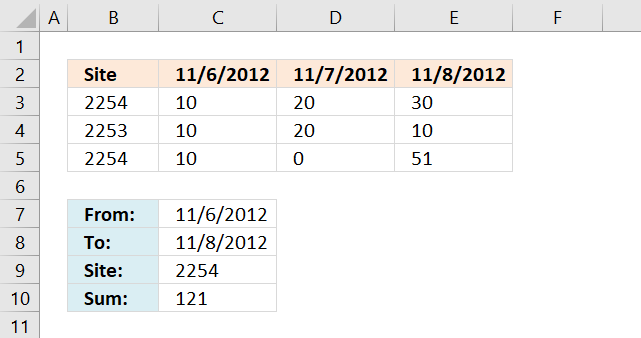Author: Oscar Cronquist Article last updated on December 07, 2018In this post, I will provide a formula to sum values in column (Qty) where a column (Date) meets two date criteria and an additional criterion in an adjacent column (Product).

I have colored the cells in column Qty that meet all criteria.

Excel formula in C18:

=SUMIFS(D3:D10, C3:C10, "="&C15, B3:B10, "<="&C14, B3:B10, ">="&C13) + ENTER

The SUMIFS function adds numbers based on a condition or criteria and returns a total.

SUMIFS(sum_range, criteria_range1, criteria1, [criteria_range2], [criteria2], ...)

The sum_range contains the numbers to be added: D3:D10

criteria_range1 (C3:C10) is the cell range that the criteria1 ("="&C15) will be applied to.

criteria_range2 (B3:B10) is the cell range (dates) that the criteria2 ("<="&C14) will be applied to.

criteria_range3 (B3:B10) is the cell range (dates) that the criteria3 (">="&C13) will be applied to.

SUMIFS(D3:D10, C3:C10, "="&C15, B3:B10, "<="&C14, B3:B10, ">="&C13)

Alternative formula in C19:

=SUMPRODUCT(--(B3:B10<=C14), --(B3:B10>=C13), --(C3:C10=C15), D3:D10) + ENTER

The SUMIFS function was introduced in Excel 2007, the SUMPRODUCT function works in all Excel versions.

Recommended post

Sum cells with check boxes

I will now demonstrate with the following table how to add check-boxes and sum enabled check-boxes using a formula. Add […]

Sum number based on corresponding unique value

The formula in cell E14 adds a number from column C if the corresponding value in column B is unique […]

### Download excel file for this tutorial

Sum values between two dates with criteria.xls
(Excel 97-2003 Workbook *.xls)

Functions in formulas above

How to use the SUM function

The SUM function in Excel allows you to add values, the function returns the sum in the cell it is […]

How to use the SUMPRODUCT function

The SUMPRODUCT function calculates the product of corresponding values and then returns the sum of each multiplication.

Site 6-Nov-12 7-Nov-12 8-Nov-12
2254 10 20 30
2253 10 20 10
2254 10 0 51
Criteria: required 2254 sum of values b/w my specified date let say from 6-8 Nov.Formula in cell C10:

=SUMPRODUCT(((\$C\$7<=\$C\$2:\$E\$2)*(\$C\$8>=\$C\$2:\$E\$2))*(\$B\$3:\$B\$5=\$C\$9)*\$C\$3:\$E\$5)